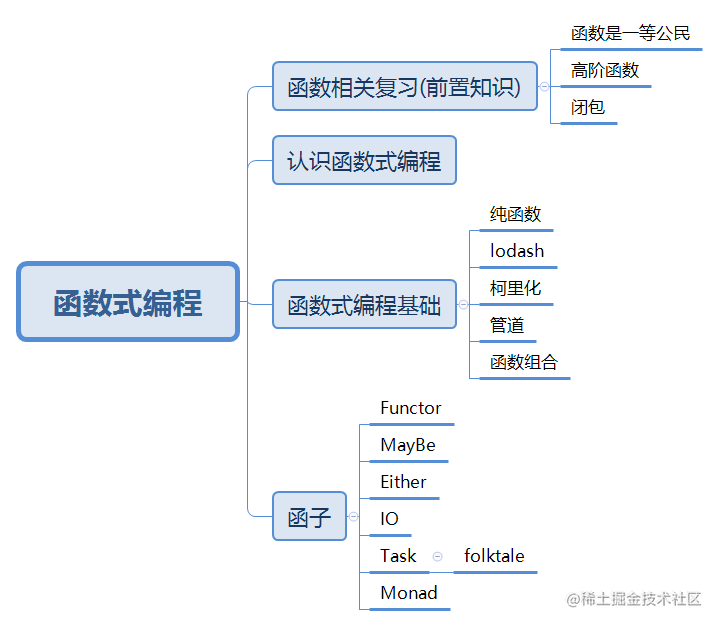# 函数式编程（一）—— 前置知识

8/19/2020 JavaScriptFunctor

## # 函数式编程总体设计

ps:对整体不太了解的先看有线函数式编程总体设计## # 为什么要学函数式编程？

• 函数式表成是随着React的流行收到越来越多的关注（React的高阶组件使用了高阶函数来实现，高阶函数就是函数式编程的一个特性。Redux也使用了函数式编程的思想。）
• Vue3也开始拥抱函数式编程
• 函数式编程可以抛弃this
• 打包过程中可以更好的利用tree shaking过滤无用代码
• 方便测试、方便并行处理
• 有很多库可以帮助我们进行函数式开发：lodash、underscore、ramda

## # 什么是函数式编程？

### # 函数式编程和面向对象编程的不同

###对于函数式编程思维方式的理解：

• 程序的本质：根据输入通过某种运算获得相应的输出，程序开发过程中会涉及很多输入和输出的函数。
• 函数式编程中的函数指的不是程序中的函数Function，而是数学中的函数即映射关系，例如：y=sin(x)，是这种x和y的关系
• 相同的输入时钟要得到相同的输出（纯函数）
• 函数式编程用描述数据（函数）之间的映射
``````// 非函数式
let num1 = 2
let num2 = 3
let sum = num1 + num2
console.log(sum)

// 函数式
function add(n1, n2) {
return n1 + n2
}
let sum = add(2, 3)
console.log(sum)

``````
1
2
3
4
5
6
7
8
9
10
11
12
13

## # 函数式编程的前置知识

### # 函数是一等公民

• 函数可以存储在变量中
``````// 把函数赋值给变量
let fn = function () {
console.log("hi")
}

fn()

// 一个示例
const BlogController = {
index (posts) { return Views.index(posts) },
show (post) { return Views.show(post) },
create (attrs) { return Db.create(attrs) },
update (post, attrs) { return Db.update(post, attrs) },
destroy (post) { return Db.destroy(post) }
}

// 优化 赋值的是Views的index方法，不是方法的调用
const BlogController = {
index: Views.index,
show: Views.show,
create: Db.create,
update: Db.update,
destroy: Db.destroy
}

``````
1
2
3
4
5
6
7
8
9
10
11
12
13
14
15
16
17
18
19
20
21
22
23
24
25

• 函数可以作为参数
• 函数可以作为返回值

### # 高阶函数

#### # 什么是高阶函数？

• 函数可以作为参数
``````// forEach
// 定义一个遍历数组的并对每一项做处理的函数，第一个函数是一个数组，第二个参数是一个函数。
function forEach (array, fn) {
for (let i = 0; i < array.length; i++) {
fn(array[i])
}
}

// test
let arr = [1, 2, 3]
forEach(arr, item => {
item = item * 2
console.log(item) // 2 4 6
})

``````
1
2
3
4
5
6
7
8
9
10
11
12
13
14
15
``````// filter
// 遍历数组，并把满足条件的元素存储成数组，再进行返回
function filter(array, fn) {
let results = []
for (let i = 0; i < array.length; i++) {
//如果满足条件
if (fn(array[i])) {
results.push(array[i])
}
}
return results
}

// test
let arr = [1, 3, 4, 7, 8]
let result = filter(arr, item => item % 2 === 0)
console.log(result) // [4, 8]

``````
1
2
3
4
5
6
7
8
9
10
11
12
13
14
15
16
17
18
• 函数作为返回值
``````// 一个函数返回另一个函数
function makeFn () {
let msg = 'Hello function'
return function () {
console.log(msg)
}
}

// test
// 第一种调用方式
const fn = makeFn()
fn() //Hello function

// 第二种调用方式
makeFn()()///Hello function

``````
1
2
3
4
5
6
7
8
9
10
11
12
13
14
15
16
``````// once
// 让函数只执行一次

function once(fn) {
let done = false
return function() {
// 判断值有没有被执行，如果是false表示没有执行，如果是true表示已经执行过了，不必再执行
if(!done) {
done = true
// 调用fn，当前this直接传递过来，第二个参数是把fn的参数传递给return的函数
return fn.apply(this, arguments)
}
}
}

// test
let pay = once(function (money) {
console.log(`支付：\${money} RMB`)
})

pay(5) //支付：5 RMB
pay(5)
pay(5)
pay(5)
pay(5)

``````
1
2
3
4
5
6
7
8
9
10
11
12
13
14
15
16
17
18
19
20
21
22
23
24
25
26

#### # 使用高阶函数的意义

• 抽象可以帮我们屏蔽细节，我们只需要知道我们的目标和解决这类问题的函数，我们不需要关心实现的细节
• 高阶函数是用来抽象通用的问题

#### # 常用的高阶函数

• forEach
• map 对数组中的每个元素进行遍历，并处理，处理的结果放在一个新数组中返回
``````const map = (array, fn) => {
let results = []
for (const value of array) {
results.push(fn(value))
}
return results
}

// test
let arr = [1, 2, 3, 4]
arr = map(arr, v => v * v)
console.log(arr)
//

``````
1
2
3
4
5
6
7
8
9
10
11
12
13
14
• filter
• every 数组中的每一个元素是否都匹配我们指定的一个条件，如果都满足返回true，如果不满足返回false
``````const every = (array, fn) => {
let result = true
for (const value of array) {
result = fn(value)
// 如果有一个元素不满足就直接跳出循环
if (!result) {
break
}
}
return result
}

// test
let arr = [11, 12, 14]
let r = every(arr, v => v > 10)
console.log(r) // false

r = every(arr, v => v > 12)
console.log(r) // false

``````
1
2
3
4
5
6
7
8
9
10
11
12
13
14
15
16
17
18
19
20
• some 判断数组中是否有一个元素满足我们指定的条件，满足是true，都不满足为false
``````const some = (array, fn) => {
let result = false
for (const value of array) {
result = fn(value)
// 如果有一个元素不满足就直接跳出循环
if (result) {
break
}
}
return result
}

// test
let arr = [1, 3, 4, 9]
let arr1 = [1, 3, 5, 9]
let r = some(arr, v => v % 2 === 0)
console.log(r) // true
r = some(arr1, v => v % 2 === 0)
console.log(r) // false

``````
1
2
3
4
5
6
7
8
9
10
11
12
13
14
15
16
17
18
19
20
• find/findIndex
• reduce
• sort

### # 闭包

#### # 闭包的概念

• 通俗的讲：可以在另一个作用域中调用一个函数的内部函数并访问到该函数作用域中的成员

``````function makeFn () {
let msg = 'Hello function'
}
// 正常情况下，执行完makeFn，里面的变量msg会释放掉
// 但是下面的情况

function makeFn () {
let msg = 'Hello function'
return function () {
console.log(msg)
}
}
// 在上面函数中，返回了一个函数，而且在函数中还访问了原来函数内部的成员，就可以称为闭包

const fn = makeFn()
fn()
// fn为外部函数，当外部函数对内部成员有引用的时候，那么内部的成员msg就不能被释放。当我们调用fn的时候，我们就会访问到msg。

//注意的点：
//1、我们可以在另一个作用域调用makeFn的内部函数
//2、当我们调用内部函数的时候我们可以访问到内部成员

``````
1
2
3
4
5
6
7
8
9
10
11
12
13
14
15
16
17
18
19
20
21
22

#### # 闭包的本质

/解读：函数执行的时候在执行栈上，执行完毕之后从执行栈上移除，内部成员的内存被释放。但是在函数执行完毕移除之后，释放内存的时候，如果外部有引用，则内部成员的内存不能被释放。/

#### # 闭包的案例

##### # 案例一：计算一个数平方和立方的运算
``````Math.pow(4, 2)
Math.pow(5, 2)
// 后面的二次方三次方很多次重复，下面要写一个二次方三次方的函数
function makePower (power) {
return function (number) {
return Math.pow(number, power)
}
}

// 求平方
let power2 = makePower(2)
let power3 = makePower(3)

console.log(power2(4)) // 16
console.log(power2(5)) // 25
console.log(power3(4)) // 64

``````
1
2
3
4
5
6
7
8
9
10
11
12
13
14
15
16
17

##### # 案例二：计算不同级别的员工工资
``````// 假设计算员工工资的函数第一个函数传基本工资，第二个参数传绩效工资
// getSalary(12000, 2000)
// getSalary(15000, 3000)
// getSalary(15000, 4000)

// 不同级别的员工基本工资是一样的，所以我们将基本工资提取出来，之后只需要加上绩效工资
function makeSalary (base) {
return function (performance) {
return base + performance
}
}
let salaryLevel1 = makeSalary(12000)
let salaryLevel2 = makeSalary(15000)

console.log(salaryLevel1(2000)) //14000
console.log(salaryLevel2(3000)) //18000
console.log(salaryLevel2(4000)) //19000
``````
1
2
3
4
5
6
7
8
9
10
11
12
13
14
15
16
17